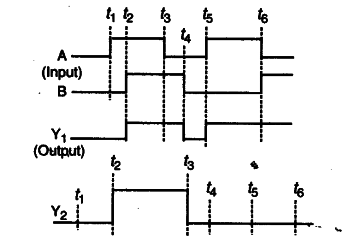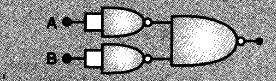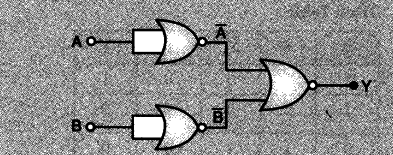# A student has to use an appropriate number of

A student has to use an appropriate number of
(i) NAND gates (only) to get the output
(ii) NOR gates (only) to get the output
From two given inputs A and B as shown in the diagram.Identify the ‘equivalent gate’ needed in each case. Show how one can connect an appropriate number of (i) NAND (ii) NOR gates respectively in the two cases to get these ‘equivalent gates’.

The equivalent gates in the two cases are the OR gate and the AND gate respectively.
(i) A combination of three NAND gates, connected in the manner shown, would be equivalent to an OR gate.(ii) A combination of three NOR gates connected in the manner shown would be equivalent to an AND gate.